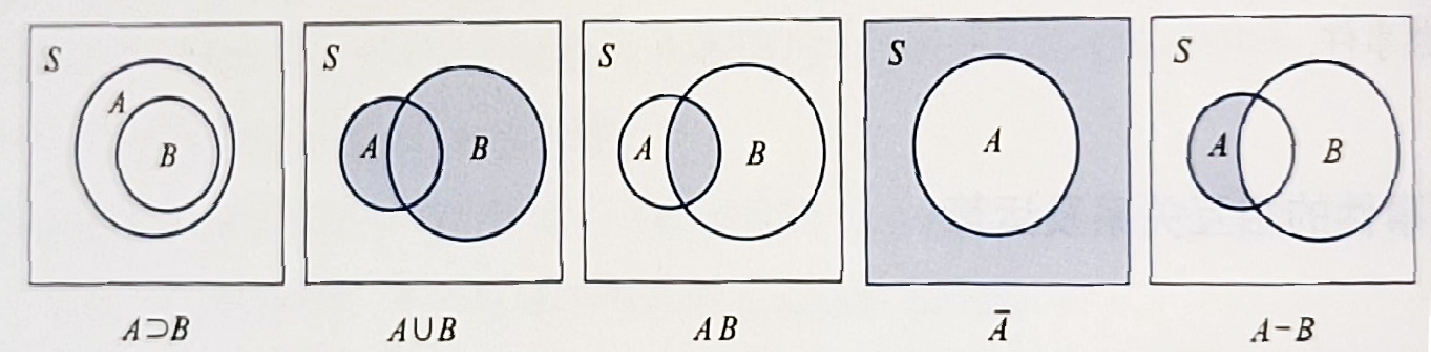# [1.x] 概率论的基本概念¶

1. 可以复现；
2. 每次试验的结果不定，但事先可以知道试验的所有可能结果；

• 特别的，只含有一个样本的子集称为基本事件从左到右分别：

• 交换律：$$A\cup B=B\cup A\;,\;A\cap B=B\cap A$$
• 结合律：$$A\cup(B\cup C)=(A\cup B)\cup C\;,\;A(BC)=(AB)C$$
• 分配律：$$A(B\cup C)=(AB)\cup(AC)\;,\;(AB)\cup C=(A\cup C)(B\cup C)$$
• 对偶律 / 德摩根定律(De Morgan's law)：$$\overline{\bigcup^n_{j=1}A_j}=\bigcap^n_{j=1}\overline{A_j}\;,\;\overline{\bigcap^n_{j=1}A_j}=\bigcup^n_{j=1}\overline{A_j}$$

• $$AB=\varnothing$$，则称两事件不相容（或互斥
• $$A\subset B \;and\;B\subset A$$，则称两事件相等

$$频率=\frac{频数}{试验总次数}$$**

1. 非负性$$P(A)\geq0$$
2. 规范性 / 正则性$$P(S)=1$$
3. 可列可加性：对于$$S$$中不相容的事件$$A_i$$，有$$P(\bigcup^{+\infty}_{j=1}A_j)=\sum_{j=1}^{+\infty}P(A_j)$$

1. 对于有限个两两不相容的事件的和事件，有$$P(\bigcup^{+\infty}_{j=1}A_j)=\sum_{j=1}^{+\infty}P(A_j)$$
2. $$P(A)=1-P(\overline A)$$
3. 特别的，可以得到$$P(\varnothing)=0$$
4. $$A\supset B$$时，$$P(A-B) = P(A)-P(B)$$$$P(A)\geq P(B)$$
5. 概率的加法公式$$P(A\cup B)=P(A)+P(B)-P(AB)$$
6. 推广即容斥原理。
7. 推论：$$P(A\cup B)\leq P(A)+P(B)$$等可能概型
如果随机事件满足：

8. $$S$$中样本点数有限；

9. $$\forall i,j \in\{1,2,...,n\},\;P(e_i) = P(e_j)$$，即等可能；

$$P(A|B)=\frac{P(AB)}{P(B)}$$ 条件概率是在新的样本空间下的概率度量，它满足概率的定义和性质。

1. $$B_iB_j=\varnothing,i,j,...,n,i\not=j$$
2. $$\bigcup^n_{i=1}B_i=S$$

$$S$$为一样本空间，$$A$$为该试验的事件，$$\{B_i\}$$$$S$$的一个划分，则有：

• $$A_1,...,A_n,...$$互不相容，则$$P(\cup_{n=1}^{\infty}A_n|B)=\sum_{n=1}^{\infty}P(A_n|B)$$
• 乘法公式：当$$P(A)\not=0\;\,\;P(B)\not=0$$时，有$$P(AB)=P(A)*P(B|A)=P(B)*P(A|B)$$
• 全概率公式$$P(A)=\sum_{j=1}^nP(B_j)P(A|B_j)$$
• 贝叶斯公式$$P(B_k|A)=\frac{P(B_kA)}{P(A)}=\frac{P(B_k)P(A|B_k)}{\sum_{j=1}^nP(B_j)P(A|B_j)}$$
• 其中，我们称$$P(B_j)$$这种事先知道的概率为先验概率；而$$P(B_j|A)$$这种，当事件$$A$$发生后需要修正$$B_j$$的概率成为后验概率

$$A,B$$为两个随机事件，若有$$P(AB)=P(A)*P(B)$$，则$$A,B$$相互独立(independent)

$$P(AB)=P(A)*P(B)\;,\;P(AC)=P(A)*P(C)\;,\;P(BC)=P(B)*P(C)$$ 都成立，则称事件$$A,B,C$$两两独立

$$P(ABC)=P(A)P(B)P(C)$$ 则称事件$$A,B,C$$相互独立

• 显然有 $$相互独立\Rightarrow两两独立$$